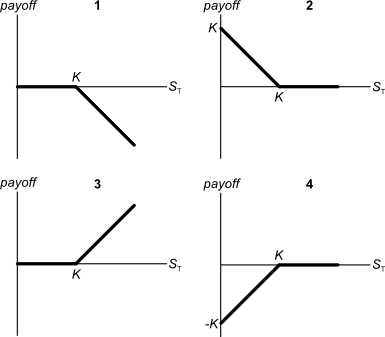# Fight Finance

#### CoursesTagsRandomAllRecentScores

Which statement about risk, required return and capital structure is the most correct?

Below are 4 option graphs. Note that the y-axis is payoff at maturity (T). What options do they depict? List them in the order that they are numberedYou have $100,000 in the bank. The bank pays interest at 10% pa, given as an effective annual rate. You wish to consume an equal amount now (t=0) and in one year (t=1) and have nothing left in the bank at the end (t=1). How much can you consume at each time? A share was bought for$4 and paid an dividend of $0.50 one year later (at t=1 year). Just after the dividend was paid, the share price fell to$3.50 (at t=1 year). What were the total return, capital return and income returns given as effective annual rates? The answer choices are given in the same order:

$r_\text{total}$, $r_\text{capital}$, $r_\text{income}$

The market expects the Reserve Bank of Australia (RBA) to increase the policy rate by 25 basis points at their next meeting.

As expected, the RBA increases the policy rate by 25 basis points.

What do you expect to happen to Australia's exchange rate in the short term? The Australian dollar will:

What is the covariance of a variable X with a constant C?

The cov(X, C) or $\sigma_{X,C}$ equals:

You owe money. Are you a or a ?

Which of the below formulas gives the payoff $(f)$ at maturity $(T)$ from being long a put option? Let the underlying asset price at maturity be $S_T$ and the exercise price be $X_T$.

Alice, Bob, Chris and Delta are traders in the futures market. The following trades occur over a single day in a newly-opened equity index future that matures in one year which the exchange just made available.

1. Alice buys a future from Bob.

2. Chris buys a future from Delta.

3. Alice buys a future from Chris.

These were the only trades made in this equity index future. What was the trading volume and what is the open interest?

Use the below information to value a levered company with constant annual perpetual cash flows from assets. The next cash flow will be generated in one year from now, so a perpetuity can be used to value this firm. Both the cash flow from assets including and excluding interest tax shields are constant (but not equal to each other).

 Data on a Levered Firm with Perpetual Cash Flows Item abbreviation Value Item full name $\text{CFFA}_\text{U}$ $48.5m Cash flow from assets excluding interest tax shields (unlevered) $\text{CFFA}_\text{L}$$50m Cash flow from assets including interest tax shields (levered) $g$ 0% pa Growth rate of cash flow from assets, levered and unlevered $\text{WACC}_\text{BeforeTax}$ 10% pa Weighted average cost of capital before tax $\text{WACC}_\text{AfterTax}$ 9.7% pa Weighted average cost of capital after tax $r_\text{D}$ 5% pa Cost of debt $r_\text{EL}$ 11.25% pa Cost of levered equity $D/V_L$ 20% pa Debt to assets ratio, where the asset value includes tax shields $t_c$ 30% Corporate tax rate

What is the value of the levered firm including interest tax shields?